150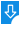10
2585

FX Equation 6.003.5 Description:
As easy it is to hand-write mathematical equations on a piece of paper, it is proportionally hard to do the exact same thing on a computer. Writing simple equations such as adding, subtracting, multiplying, and diving can easily be done using a normal, day-to-day, word processor. Despite the fact that most fully featured word-processor have built-in equation writing features, things tend to get a lot more complicated when it comes to advanced mathematical equations such as linear, Cartesian, parametric, algebraic, differential, integral, and difference, and other kinds of complex equations. FX EquationFX Equation: FX Equation for Mac, A straightforward and efficient cross-platform application that makes it easy for you to write and create equations using your Mac
Publisher: Efofex SoftwareDownload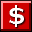Buy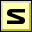Screenshot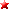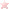Rate it
Category: Math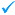Publisher: Efofex Software    More titles >>
Last Updated: 03/09/2019
Requirements: Mac OS X